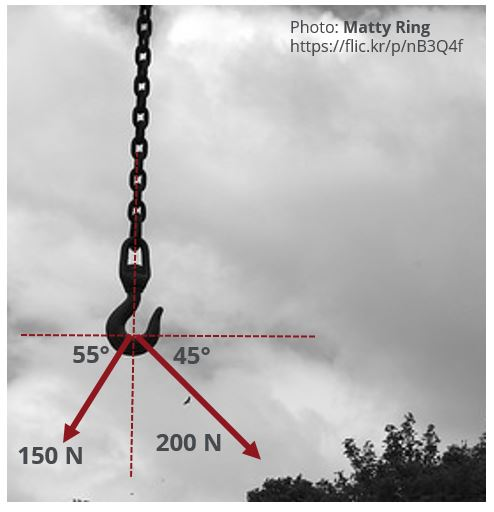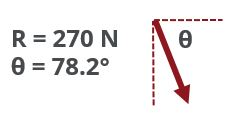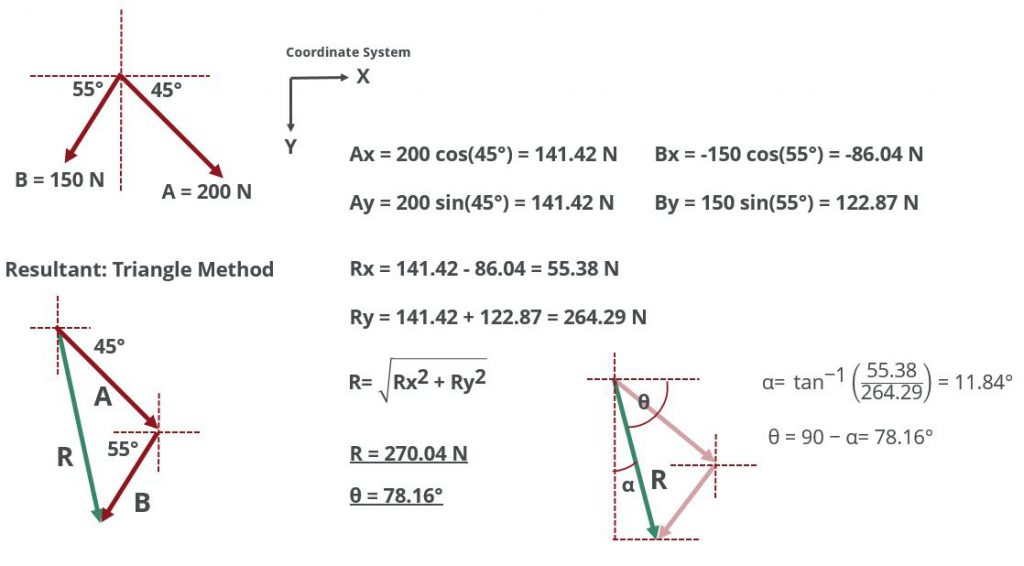# Vectors and Scalars

Andrew Wood | 28 June 2022

## Introduction

Vector and Scalar quantities are mathematical formulations that assist us in modelling the physical quantities of the world around us. Some quantities can be fully described by just quoting the quantity’s value or magnitude, for example: the temperature today is 74°F. The temperature at a point is constant at that point and doesn’t require knowing the direction to fully describe it.

However, some quantities can not be described fully by just quoting the magnitude of the quantity. The engine produces 2000 lbs of thrust may describe how much thrust is being produced, but it does not tell us in what direction the thrust force is being applied. Producing 2000 lbs of thrust vertically upward will have a very different effect to producing 2000 lbs of thrust forwards.

This leads us to the essential difference between a scalar and a vector quantity:

A scalar quantity is such a quantity that depends only on magnitude and not direction to fully describe it.

A vector quantity is a quantity that requires a magnitude and a direction to fully describe it.

Here are some common scalar and vector quantities we find in aeronautical engineering:

Scalar Vector
temperature lift
volume drag
mass weight
density thrust
area acceleration
pressure momentum
speed velocity
energy displacement
power
length

### Vector Respresentation

Vectors are visually represented by an arrow of given magnitude and direction. The length of the arrow denotes the magnitude of the vector while its direction is shown by the angle between the vector’s line of action and a given reference axis. The sense of direction is denoted by the direction of the arrowhead with the arrowhead always placed at the head of the vector.

Mathematically, a vector quantity is often represented by a letter with an arrow placed above it, like $$\vec{A}$$ or alternatively by using a boldface font like, $$\mathbf{A}$$. The magnitude of vector $$\mathbf{A}$$ is a scalar value and is represented like so, $$|\mathbf{A}|$$.

## Vector Operations

Vectors can be added or subtracted from one another in the following way:

Force vectors $$\mathbf{A}$$ and $$\mathbf{B}$$ acting on a single point may be added together to create a resultant force R using either the method of triangular or parallelogram construction:

The triangle method works by adding the tail of $$\mathbf{B}$$ to the head of $$\mathbf{A}$$. The resultant vector $$\mathbf{R}$$ is then calculated by joining the tail of $$\mathbf{A}$$ to the head of $$\mathbf{B}$$.

The parallelogram method works by joining $$\mathbf{A}$$ and $$\mathbf{B}$$ at their tails. Parallel lines to $$\mathbf{A}$$ and $$\mathbf{B}$$ are created from the heads of $$\mathbf{B}$$ and $$\mathbf{A}$$ respectively to form a parallelogram. The diagonal between the tail of $$\mathbf{A}$$ and $$\mathbf{B}$$ and the point at which the parallel lines intersect forms the resultant vector $$\mathbf{R}$$.

Both methods will produce a resultant $$\mathbf{R}$$ with equal magnitude and direction.

### Cartesian Coordinates

Often the most practical method for adding vectors together is to make use of a Cartesian coordinate system to break each vector down into a pair of orthogonal vectors that fully describe the original vector. The vector components which act on the same line of action can then be added to one another. The resultant formed by adding these vectors is of equal magnitude and direction to the resultant formed by using either the triangle or parallelogram methods.

Multiplication of a vector by a scalar has the effect of only changing the magnitude of the vector but not its line of action. However, the sense of the vector can be changed by multiplying the vector by a negative scalar.

Division of a vector by a scalar is analogous to multiplication of a vector by the inverse of the scalar.

$$\frac{\vec{A}}{a} = \vec{A}\times\frac{1}{a}$$

Now that we've covered the basics of vector mathematics let's dive in an try an example. Try and go through the example yourself first before revealing the answer and working.#### Calculate the magnitude and direction of the resultant vector.Share
Category
Tags